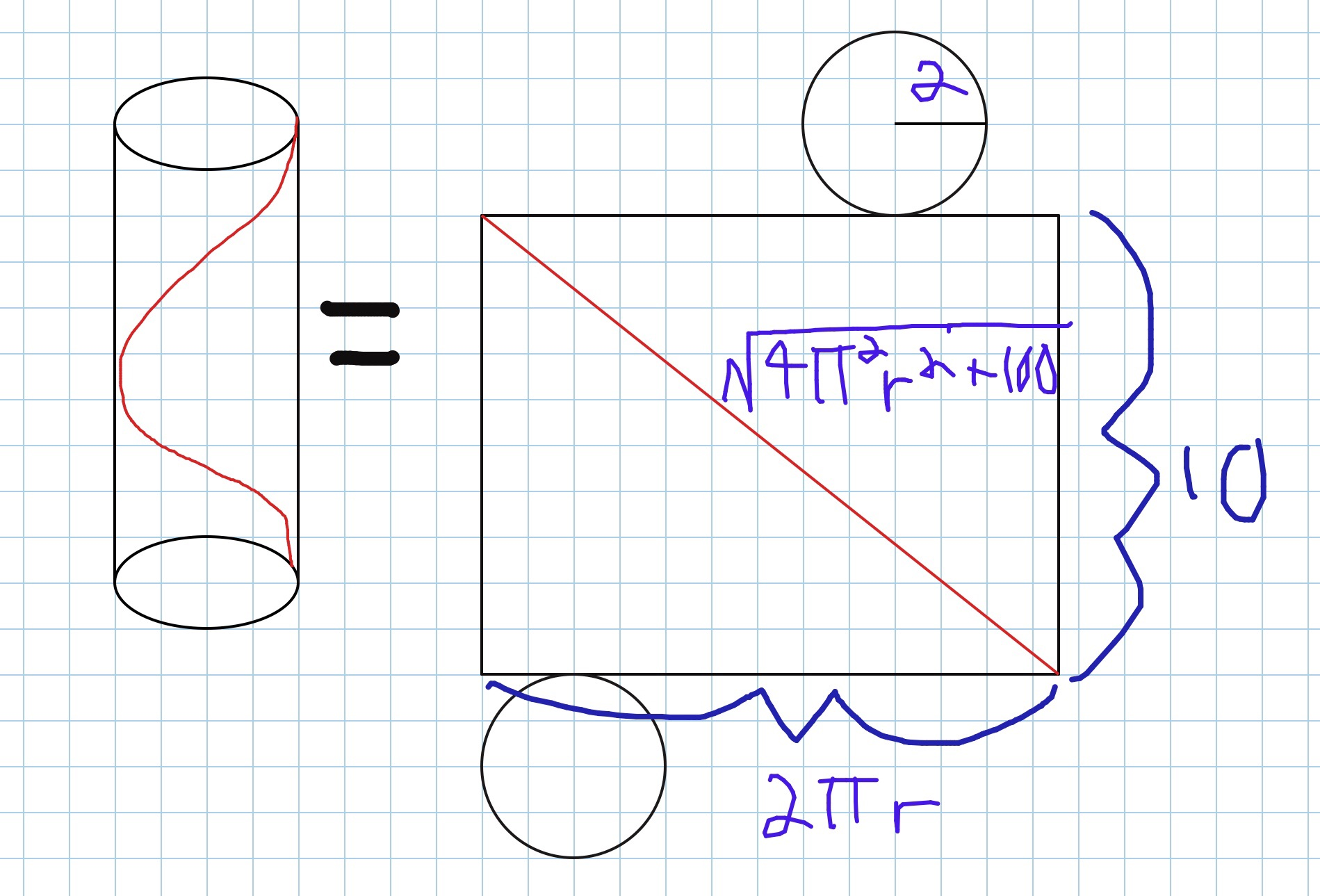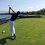# Generalized length of 3D Cylindrical spiral: NO CALC NEEDED!In one of my previous problems, I posted finding the length of a spiral stair case. I always wondered how to find the length of a 3D spiral if every segment was congruent to every other. I never thought to visualize it as a cylinder.

Above, you can see the spiral and the cylinder's net. The height of the cylinder is the height of the rectangle and the circumference of the circle is the width. By the pythagorean theorem, the length of one spiral will be $\sqrt{4\pi^2r^2+h^2}$.

Now, since all parts are congruent, we can multiply it by the degree of turning (1 spiral =360 or $2\pi$) relative to one turn. Tus our final formula is $\sqrt{4\pi^2r^2+h^2}\left(\frac{\theta}{360}\right)$ where $\theta$ is the degree of turning.Note by Trevor Arashiro
6 years, 9 months ago

This discussion board is a place to discuss our Daily Challenges and the math and science related to those challenges. Explanations are more than just a solution — they should explain the steps and thinking strategies that you used to obtain the solution. Comments should further the discussion of math and science.

When posting on Brilliant:

• Use the emojis to react to an explanation, whether you're congratulating a job well done , or just really confused .
• Ask specific questions about the challenge or the steps in somebody's explanation. Well-posed questions can add a lot to the discussion, but posting "I don't understand!" doesn't help anyone.
• Try to contribute something new to the discussion, whether it is an extension, generalization or other idea related to the challenge.

MarkdownAppears as
*italics* or _italics_ italics
**bold** or __bold__ bold
- bulleted- list
• bulleted
• list
1. numbered2. list
1. numbered
2. list
Note: you must add a full line of space before and after lists for them to show up correctly
paragraph 1paragraph 2

paragraph 1

paragraph 2

[example link](https://brilliant.org)example link
> This is a quote
This is a quote
    # I indented these lines
# 4 spaces, and now they show
# up as a code block.

print "hello world"
# I indented these lines
# 4 spaces, and now they show
# up as a code block.

print "hello world"
MathAppears as
Remember to wrap math in $$ ... $$ or $ ... $ to ensure proper formatting.
2 \times 3 $2 \times 3$
2^{34} $2^{34}$
a_{i-1} $a_{i-1}$
\frac{2}{3} $\frac{2}{3}$
\sqrt{2} $\sqrt{2}$
\sum_{i=1}^3 $\sum_{i=1}^3$
\sin \theta $\sin \theta$
\boxed{123} $\boxed{123}$

Sort by:

And this is why we love math. Thanks for sharing.

Staff - 6 years, 9 months ago

Wow, thank you so much. That means a lot to me. :D

- 6 years, 9 months ago

Nice.... Keep it up dude..

- 6 years, 9 months ago

Great!

- 6 years, 9 months ago

Too cool!!!thanks for sharing.totally loved it

- 6 years, 9 months ago

- 6 years, 9 months ago

Awesom man . ..

- 6 years, 6 months ago